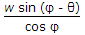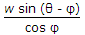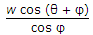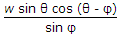# Civil Engineering - Applied Mechanics

### Exercise :: Applied Mechanics - Section 4

36.

If a spherical body is symmetrical about its perpendicular axes, the moment of inertia of the body about an axis passing through its centre of gravity as given by Routh's rule is obtained by dividing the product of the mass and the sum of the squares of two semi-axes by n where n is

 A. 2 B. 3 C. 4 D. 5

Explanation:

No answer description available for this question. Let us discuss.

37.

The angle of projection for a range is equal to the distance through which the particle would have fallen in order to acquire a velocity equal to the velocity of projection, will be

 A. 30° B. 45° C. 60° D. 75°.

Explanation:

No answer description available for this question. Let us discuss.

38.

A particle executes a simple harmonic motion. While passing through the mean position, the particle possesses

 A. maximum kinetic energy and minimum potential energy B. maximum kinetic energy and maximum potential energy C. minimum kinetic energy and maximum potential energy D. minimum kinetic, energy and minimum potential energy E. none of these.

Explanation:

No answer description available for this question. Let us discuss.

39.

A body of weight w placed on an inclined plane is acted upon by a force P parallel to the plane which causes the body just to move up the plane. If the angle of inclination of the plane is θ and angle of friction is φ, the minimum value of P, is

 A.B.C.D.Explanation:

No answer description available for this question. Let us discuss.

40.

Varigon's theorem of moments states

 A. arithmetical sum of the moments of two forces about any point, is equal to the moments of their resultant about that point B. algebraic sum of the moments of two forces about any point, is equal to the moment of their resultant about that point C. arithmetical sum of the moments of the forces about any point in their plane, is equal to the moment of their resultant about that point D. algebraic sum of the moments of the forces about any point in their plane, is equal to the moment of their resulant about that point.